Theory Which Invalidates Itself
Einstein's “Theory'' of Relativity Must be Removed from Science
 Shortest Debunking of Einstein's Relativityinstein's “theory'' of relativity is so obviously wrong that no time and effort should be wasted to discuss it, thus giving it legitimacy. On the other hand, in view of the high extent of damage to the public interest, the removal of Einstein's “theory'' of relativity from physics must be the top priority and a matter of highest responsibility and urgency for every scientist worth his or her salt. It would be impossible to carry out any other scientific research, knowing that the so-called “big-science'' (high-energy physics, particle physics, cosmology, string theories, dark matter, black holes, Big Bang etc.) is occupied by bad science, having as its fundamentals Einstein's “theory'' of relativity. Removal from science of the non-physical Einstein's “theory'' of relativity will also require removal from science of all the above “big-science'' areas as well as deeply entrenched incorrect ideas about curvature of space, space-time and anything else physically wrong that these arise from, such as Lorentz transformations. In this way very substantial societal resources will be freed from being occupied by junk science, entrenched on a staggering scale. Consequently, resources will be redirected to truly productive scientific research, in compliance with reality. One-liners are just enough to prove it invalid and the fun part and the race is how to make the damning argument as short as possible, while using its own devices and not even seeking for paradoxes outside of it, no matter how legitimate those paradoxes might be to debunk that “theory''. Here are several short ways to do it, each one quite enough in its own right to overthrow it completely, accompanied by short study guides for those willing to waste their time on the origins of something senseless but pushed to undeserved prominence. Spatially Coinciding Clocks Are Synchronous The time in a given place at a given moment in a given system has only one single value, independent of whether said time is measured by a clock K at rest in that place or said time is measured by a moving clock k, which happens to be in that place at that moment.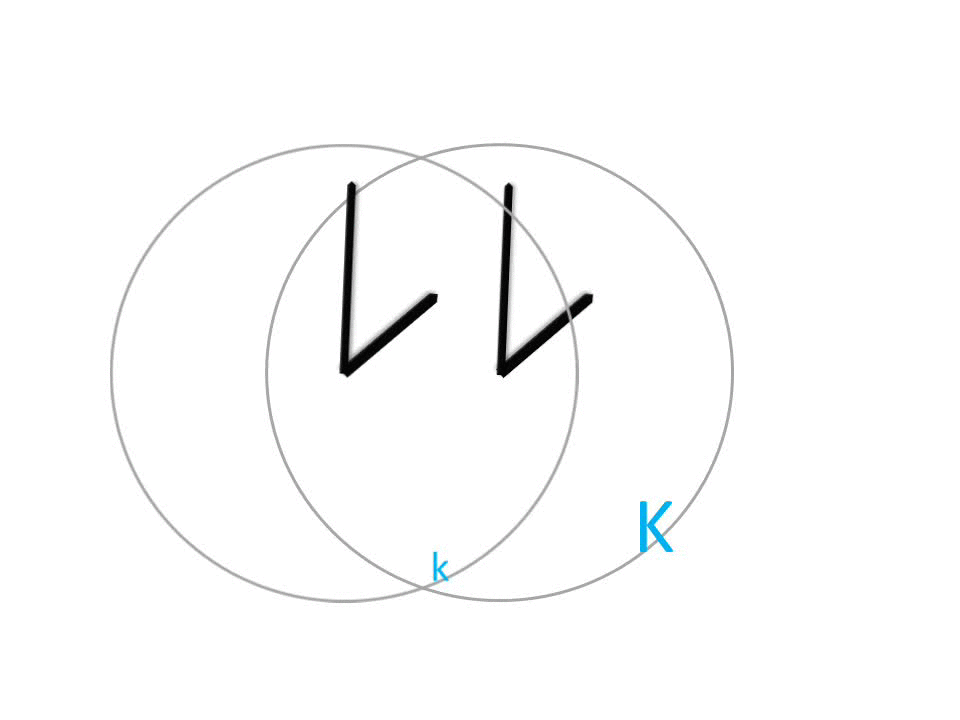The above-stated absolute truth should be enough to uderstand that the rate of time is the same in all systems and that time-dilation (clocks running at different rate of time when moving) is impossible in principle. Moving clocks cannot show that the time at a given place is different from what it really is in that place at that moment. Thus, whatever corrections there may be in GPS, these corrections cannot at all be due to variations in time rate, falsely claimed by Einstein's “theory'' of relativity. Clearly, it is out of the question that these GPS corrections be used as a proof for the validity of Einstein's “theory'' of relativity. Furthermore, in view of the above conclusive argument, any claim whatsoever for experimental confirmation of time-dilation must necessarily be considered either an experimental error or deliberately misleading. Physics must return to the only possible understanding of time that has basis in reality; namely, that time in the Universe runs at the same rate everywhere‒‒in a given system, as well as, in any other system, moving with respect to that system. Time is absolute. The above invalidates at once Einstein's “theory'' of relativity in its entirety and mandates that it be removed from physics without delay. Non-Physical Second Postulate It should not be hard to understand that the second postulate of Einstein's “theory'' of relativity is obviously physically impossible because of the just stated absolute fact that two spatially coinciding clocks are synchronous (a fact recognized by Einstein himself, by the way, but not heeded by him in building his “theory'').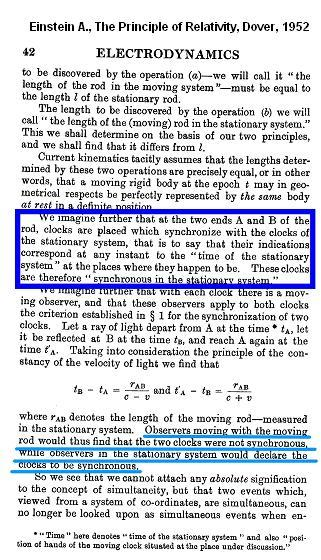Thus, all clocks in K are synchronous at all moments (if you are not sure that this is the case click here). Because, as emphasized above, all spatially coinciding clocks are synchronous, then all clocks attached permanently to a moving rigid body k (moving with respect to K) are also synchronous with those already talked about synchronous clocks in K. Note that there may be as many clocks permanently attached to the moving rigid body k as one wishes. Since every one of these permanently attached clocks individually is synchronous with the synchronous clocks in K, then these permanently attached clocks are synchronous among themselves in K, as well. The permanently attached clocks to the moving body k are also synchronous among themselves in k because these are exactly the same clocks under consideration. Motion does not make a given clock moving in K different from the same clock in its own system k Let us repeat the above conclusion: All clocks in the stationary system K are synchronous. All clocks in the moving system k are also synchronous, even if viewed from system with respect to which they are moving. Therefore, Einstein is wrong in concluding that synchronous clocks in one system will be viewed as asynchronous from another system, moving with respect to the first. Simultaneity is absolute. The above leads to nothing less than realizing that, as already said, the second postulate is physically impossible. Indeed, the time of flight of a light ray from one clock to another clock in k and then back, cannot be different, as Einstein derives for the clocks in k because, as seen, all clocks in k are synchronous. Make some effort to understand the undeniable physical fact that the inequality of the times of light ray flight derived by Einstein can only be true for synchronous clocks, as the clocks in k are, if the light has different velocities in the two direction. This obliterates at once the second postulate. Lorentz Transformations Destroy the Concept of Length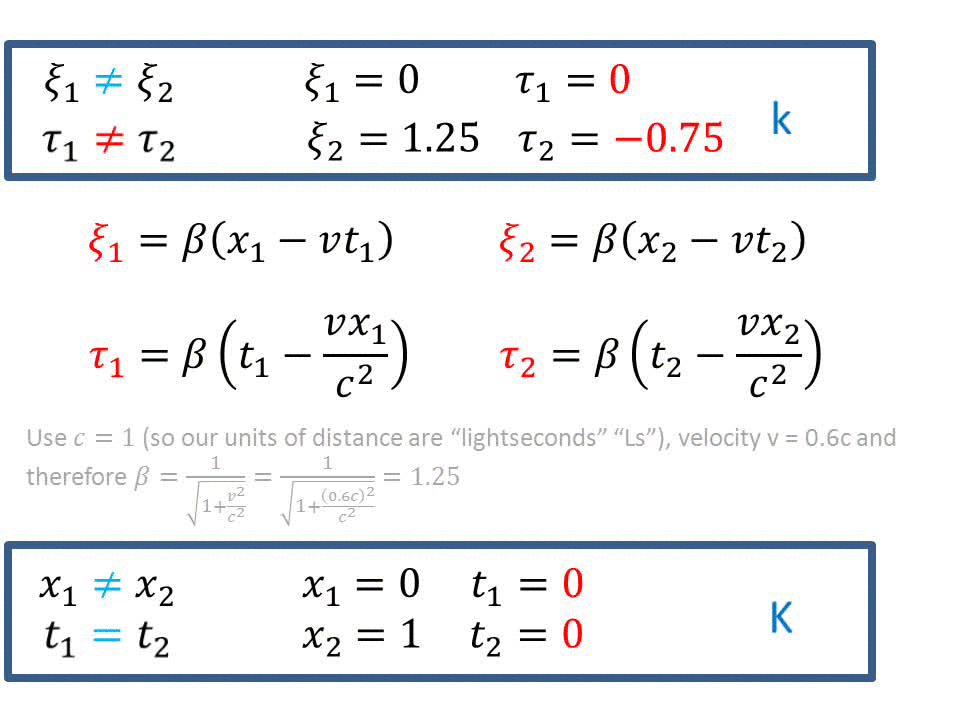Lorentz transformations represent an intact rigid body in stationary system K as a disjointed body in moving system k, with no two points of that body existing at the same time. By definition, length of the body in K is x2 - x1 because both these points exist at time t1 together. It is, however, impossible to speak about length of that same body in k because the coordinates ξ1 and ξ2, calculated by the Lorentz transformations, do not even exist at the same time; as seen, the corresponding times of their existence, τ1 and τ2, are different. Therefore, claiming that there might be length-contraction, as Einstein's “theory'' of relativity does, is out of the question. What length-contraction since the very concept of length is non-existent after the application of the Lorentz transformations? Internal Contradictions It is seen at once that Enstein's “theory'' of relativity derives two completely different expressions for one and the same law, which refers to a given unique single body in a given single system.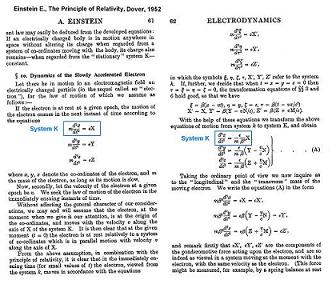Newton's second law is derived to be both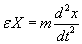and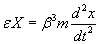for one and the same unique electron in the given single system K. The student, curious to learn the origins of such manipulation, which was most brazenly passed for science, may want to follow this link to a study guide or this link to a study guide. An analogy may be useful for those who fear formuli. The “theory'' presented by Einstein is akin to a following ingenious “theory'': Let us be given a male who is alive at all times. Let us also be given that at the same time this same male be dead at all times. Now, having postulated these two initial conditions, the great discoverer and genius first conveniently remembers only one of these initial conditions whereby it was postulated that the male is dead at all times (the genius, being a true genius, deliberately forgets at this point that, according to the other initial condition, the male is alive at all times). However, if that is true; that is, if the given male is dead at all times, then there is no living male. On the other hand, the genius reasons, according to the initial conditions, there is a living person at all times and, in general, a living person can be nothing other than either a male or a female (intersex individuals excluded in this argument). However, it was just concluded that there is no living male. Therefore, the discussed living person must be a female! In other words, a seminal discovery is made that a male from one perspective is a female from another. Sex is relative. Does a sane person need an explanation as to why the above “theory'' is nothing else but outright gibberish? Einstein's “Theory'' of Relativity Has Nothing to Do With E = mc2 The above crucial aguments are quite enough to convince the diligent student that it is out of the question to claim that Einstein's “theory'' of relativity has anything to do with E = mc2. If one still needs proof that Einstein's “theory'' of relativity has nothing to do with E = mc2, here is a purely formal demonstration. Thus, observe that Einstein confuses the constant velocity v with which system k is traveling with respect to K, and which is a velocity, which is not a function of x,on the one hand, and the velocity v, function of x, whose first derivative constitutes part of the constant force Fx, applied at time t = 0, on the other hand. Notice, that latter velocity; namely, velocity v, would not even be a subject of discussion, had the constant force not been applied; constant velocity v being then the only velocity that one can speak of.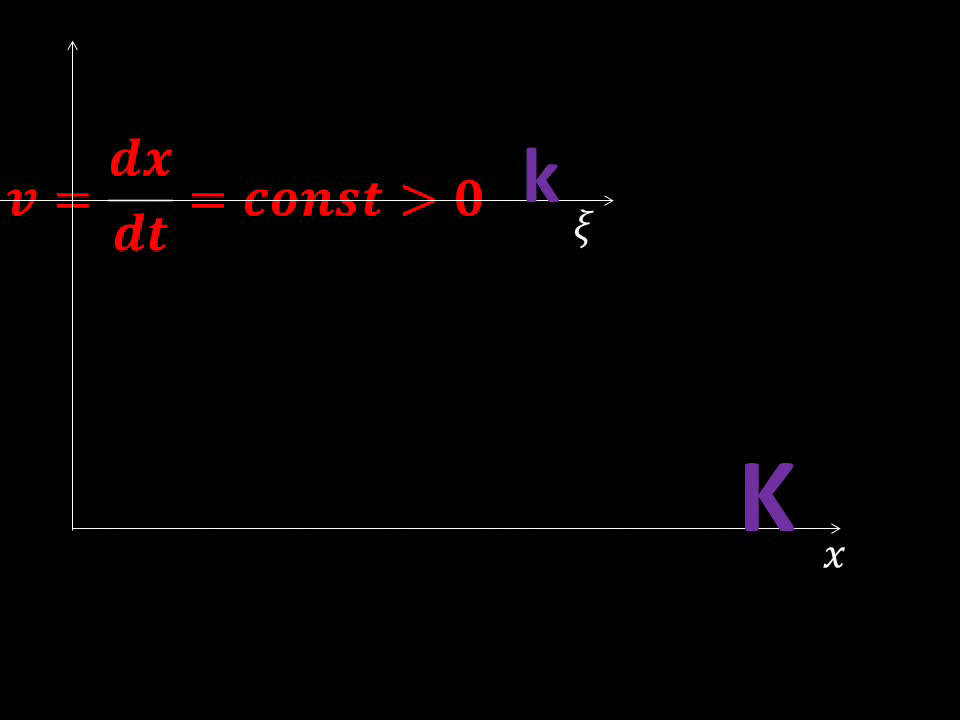Constant velocity v leads to the constancy of coefficient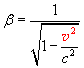, allowing to factor it out of the integral, as seen in the gif below. Thus, the most Einstein has achieved is to deriving a wrong formula for the kinetic energy in K. Most importantly, the wrong formula for the kinetic energy in K has nothing to do whatsoever with deriving E = mc2, clearly demonstrating that Einstein has nothing to do with E = mc2 but his “theory'' cannot even derive that so much celebratedly ascribed to Einstein mass-energy relationship.E = mc2 is inherent in classical physics. Recurrent Flaw in Thinking Seen in Another Einstein Derivation, Unrelated to Einstein's “Theory'' of Relativity It turns out, astonishing as it may sound, that the disregard of logic and of elementary absolute truths, such as the uniqueness of a given body and the laws that govern it in a given system, is not accidental only regarding Einstein's “theory'' of relativity. The careful student will undoubtedly find with great amazement, that the same defective thinking, in defiance of inevitable absolute truths involving uniqueness, is applied in another, unrelated, study by the same author: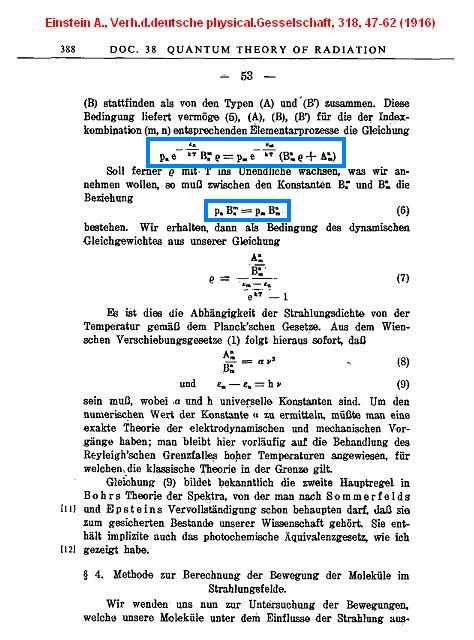In the text shown above, we see an equilibrium equation, corresponding to lower temperature T1On the other hand, the same equilibrium equation appropriates the following form at higher temperature T2: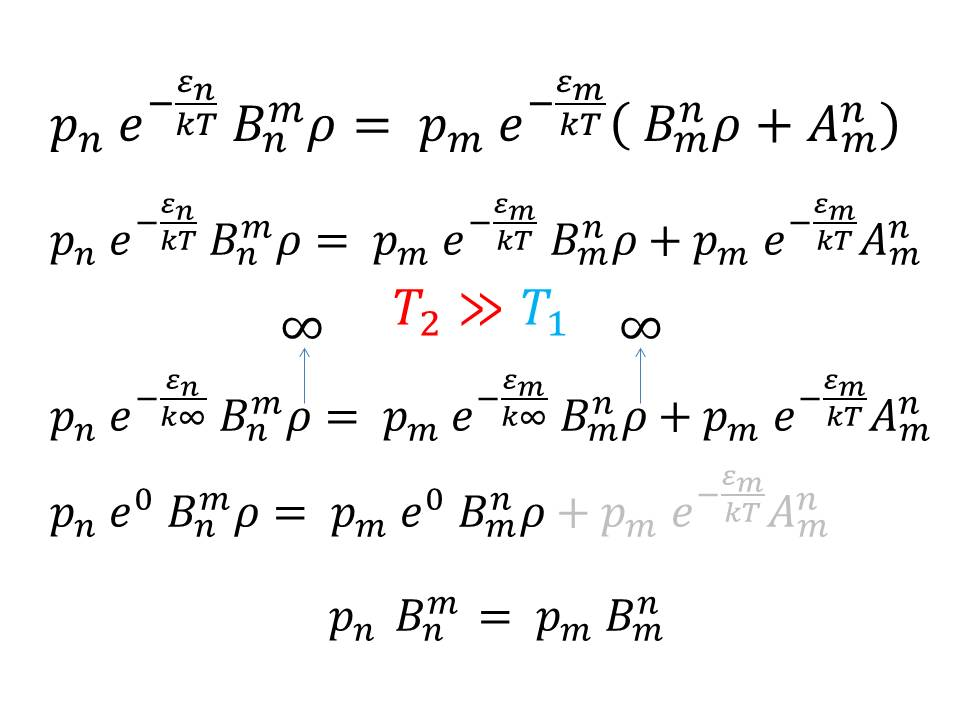And here is the blatant error. Einstein's substitution of pn, derived from an equation, corresponding to higher temperature T2, into the equation, corresponding to the lower temperature T1, is clearly unacceptable, despite the fact that the result resembles Planck's radiation law. At a given temperature, a given equilibrium can only be expressed by one single equation and not by two different equations, as Einstein incorrectly foists on us.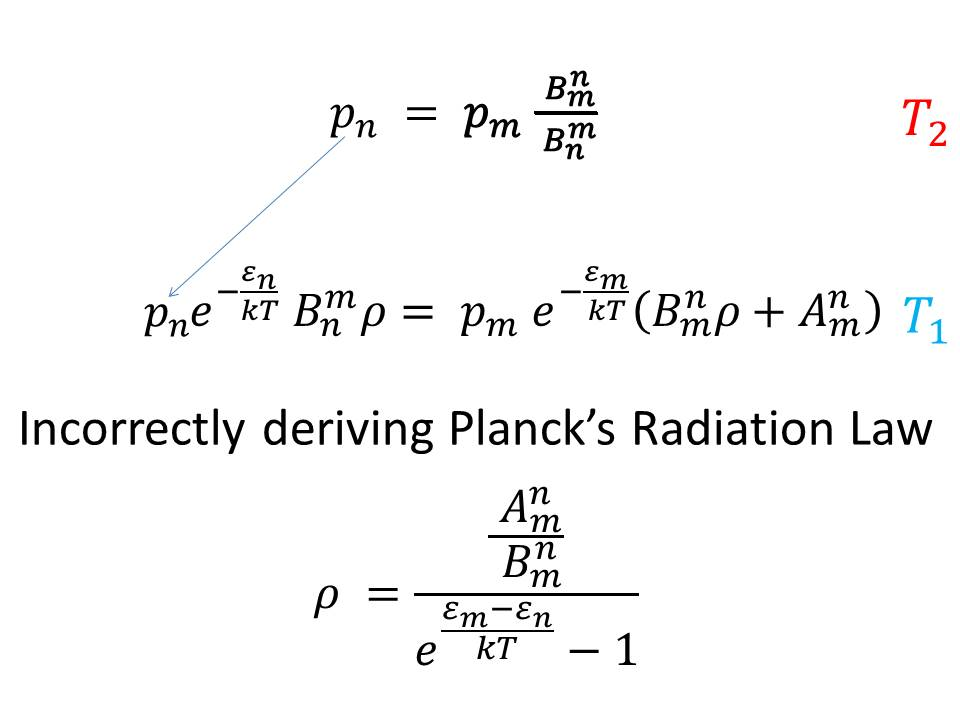Thus, the student must be instructed in as plain language as possible, that physics is not only burdened with the bad science of Einstein's “theory'' of relativity, which mandates prompt removal in its entirety, including its various applications such as cosmology, string theories, participation in electrodynamics, particle physics and so on, but also lacks a correct theory of lasers. A correct theory of lasers must not have in its basis senselessness, passed as derivation, such as that shown above. Unfortunately, the above-shown senselessness, is the currently used starting point to explain laser action. Such low quality thinking is not the thinking of a genius, which is the least conclusion that can be made from the above. The main conclusion is that Einstein's “theory of relativity must be removed from science. E = mc2 in Ampere's Law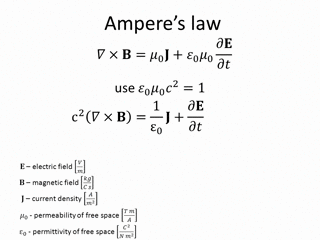email of the author:: timeisabsolute@outlook.com